## Bauer's Identical Congruence

Letdenote the set of thenumbers less than and Relatively Prime to, whereis the Totient Function. Define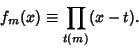(1)

A theorem of Lagrange states that(2)

This can be generalized as follows. Let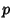be an Odd Prime Divisor ofand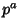the highest Power which divides, then(3)

and, in particular,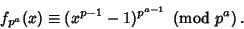(4)

Furthermore, if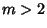is Even and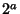is the highest Power of 2 that divides, then(5)

and, in particular,(6)

Hardy, G. H. and Wright, E. M. Bauer's Identical Congruence.'' §8.5 in An Introduction to the Theory of Numbers, 5th ed. Oxford, England: Clarendon Press, pp. 98-100, 1979.